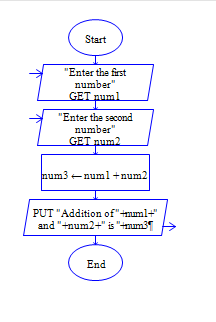Python program to get two integer numbers, add both the integers and display the sum.

Sample Input 1:

5 6

Sample Output 1:

11

#### Flow Chart Design#### Program or Solution

``` num1=int(input("Enter First number:")) num2=int(input("Enter second number:")) sum1=num1+num2 print(sum1) ```

#### Program Explanation

Read two inputs using input() method.

Conver it into integer by int() method.